# 平行线和垂直线

## 平行线

 坡度是直线方程里的 m 值： y = mx + b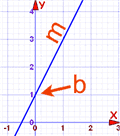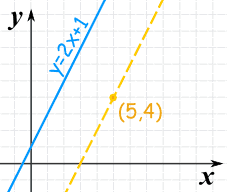### 例子：

• y = 2x + 1 平行
• 经过 (5,4) 这点

y=2x+1 的坡度是：2

y − y1 = 2(x − x1)

y − 4 = 2(x − 5)

y − 4 = 2x − 10

y = 2x − 6

### 例子：y = 3x + 2 与 y − 2 = 3x 平行吗？

y = 3x + 2：坡度是 3，Y截距是 2

y − 2 = 3x：坡度是 3，Y截距是 2

## 垂直线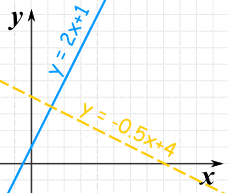线 坡度 y = 2x + 1 2 y = −0.5x + 4 −0.5

2 × (−0.5) = −1

### 应用

m1m2 = −1

 也可以写成： m1 = −1/m2 或 m2 = −1/m1

• 计算 1/坡度 （倒数
• 然后取计算结果的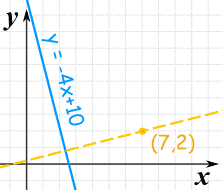### 例子：

• y = −4x + 10 垂直,
• 并穿过 (7,2) 这点

• 的线的方程

y=−4x+10 的坡度是：−4

m = − 1−4 = 14

y − y1 = (1/4)(x − x1)

y − 2 = (1/4)(x − 7)

y − 2 = x/4 − 7/4

y = x/4 + 1/4

## 垂直线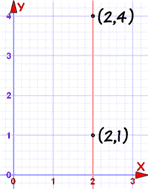m = yA − yBxA − xB = 4 − 12 − 2 = 30 = 未定义

• 两条垂直线互相平行。
• 垂直线与水平线互相垂直。